StatLect

# Hypothesis testing

Hypothesis testing is a method of making statistical inferences in which:

• we establish an hypothesis, called null hypothesis;

• we use some data to decide whether to reject or not to reject the hypothesis.

This lecture provides a rigorous introduction to the mathematics of hypothesis tests, and it provides several links to other pages where the single steps of a test of hypothesis can be studied in more detail.## What you need to know to get started

Remember that a statistical inference is a statement about the probability distribution from which a sample has been drawn.

In mathematical terms, the samplecan be regarded as a realization of a random vector, whose unknown joint distribution functionis assumed to belong to a set of distribution functions, called statistical model.

Example We observe the realizationsofindependently and identically distributed (IID) random variables having a normal distribution. The sample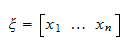can be regarded as a realization of a random vectorwhose entries are all independent of each other. The statistical model is a set of distribution functions satisfying certain conditions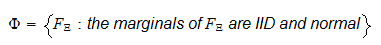We will continue this example in the following sections.

## Testing restrictions

In hypothesis testing we make a statement about a model restriction involving a subsetof the original model.

The statement we make is chosen between two possible statements:

1. reject the restriction;

2. do not reject the restriction.

Roughly speaking, we start from a large setof distributions that might possibly have generated the sampleand we would like to restrict our attention to a smaller set.

In a test of hypothesis, we use the sampleto decide whether or not to indeed restrict our attention to the smaller set.

Example In the case of our normal sample, we might want to test the restriction that the mean of the distribution is equal to zero. The restriction would be: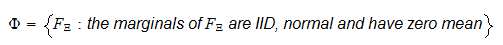## Parametric tests

Remember that in a parametric model the set of distribution functionsis put into correspondence with a setof-dimensional real vectors called the parameter space.

The elements ofare called parameters and the true parameter is denoted by. The true parameter is the parameter associated with the unknown distribution functionfrom which the samplewas actually drawn. For simplicity,is assumed to be unique.

In parametric hypothesis testing we have a restrictionon the parameter space and we choose one of the following two statements about the restriction:

1. reject the restriction;

2. do not reject the restriction.

For concreteness, we will focus on parametric hypothesis testing in this lecture, but most of the things we will say apply with straightforward modifications to hypothesis testing in general.

Example In the above example, a normal distribution is completely described by its meanand variance. Thus, each distribution in the setis put into correspondence with a parameter vector. In this case the parameter space is. The restriction to be tested is that the mean of the distribution be equal to zero. Therefore, the parametric restriction is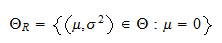## Null hypothesis

The hypothesis that the restriction is true is called null hypothesis and it is usually denoted by:Understanding how to formulate a null hypothesis is a fundamental step in hypothesis testing. We suggest to read a thorough discussion of null hypotheses here.

Example In our example, the null hypothesis is## Alternative hypothesis

The restriction(whereis the complement of) is often called alternative hypothesis and it is denoted by:Statisticians sometimes take into consideration as an alternative hypothesis a set smaller than. In these cases, the null hypothesis and the alternative hypothesis do not cover all the possibilities contemplated by the parameter space.

For some authors, "rejecting the null hypothesis" and "accepting the alternative hypothesis" are synonyms. For other authors, however, "rejecting the null hypothesis" does not necessarily imply "accepting the alternative hypothesis".

Although this is mostly a matter of language, it is possible to envision situations in which, after rejecting, a second test of hypothesis is performed wherebybecomes the new null hypothesis and it is rejected (this may happen for example if the model is mis-specified).

In these situations, if "rejecting the null hypothesis" and "accepting the alternative hypothesis" are treated as synonyms, then some confusion arises, because the first test leads to "accept" and the second test leads to "reject".

Example In our example, the alternative hypothesis could be## Types of errors

When we decide whether to reject a restriction or not to reject it, we can incur in two types of errors:

1. reject the restrictionwhen the restriction is true; this is called an error of the first kind or a Type I error;

2. do not reject the restrictionwhen the restriction is false; this is called an error of the second kind or a Type II error.

Example In our example, if we reject the restrictionwhen it is true, we commit a Type I error.

## Critical region

Remember that the sampleis regarded as a realization of a random vectorhaving support.

A test of hypothesis is usually carried out by explicitly or implicitly subdividing the supportinto two disjoint subsets.

One of the two subsets, denoted byis called the critical region (or rejection region) and it is the set of all values offor which the null hypothesis is rejected: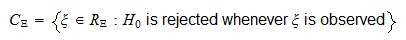The other subset is the complement of the critical region: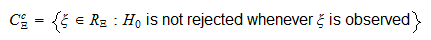and it is, of course, such that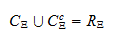This mathematical formulation is made more concrete in the next section.

## Test statistic

The critical region is often implicitly defined in terms of a test statistic and a critical region for the test statistic.

A test statistic is a random variablewhose realization is a function of the sample.

A critical region foris a subsetof the set of real numbers and the test is performed based on the test statistic, as follows: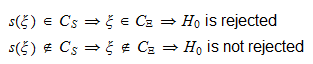If the complement of the critical regionis an interval, then its extremes are called critical values of the test. See this glossary entry for more details about critical values.

Example In our example, where we are testing that the mean of the normal distribution is zero, we could use a test statistic called z-statistic. If you want to read the details, go to the lecture on hypothesis tests about the mean.

## Power function

The power function of a test of hypothesis is the function that associates the probability of rejectingto each parameter.

Denote the critical region by.

The power functionis defined as follows: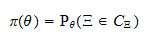where the notationis used to indicate the fact that the probability is calculated using the distribution functionassociated to the parameter.

## Size of a test

When, the power functiongives us the probability of committing a Type I error, that is, the probability of rejecting the null hypothesis when the null hypothesis is true.

The maximum probability of committing a Type I error is, therefore,This maximum probability is called the size of the test.

The size of the test is also called by some authors the level of significance of the test. However, according to other authors, who assign a slightly different meaning to the term, the level of significance of a test is an upper bound on the size of the test.

In mathematical tems, the level of significance is a constantthat, to the statistician's knowledge, satisfies## Criteria to evaluate tests

Tests of hypothesis are most commonly evaluated based on their size and power.

An ideal test should have:

• size equal to(i.e., the probability of rejecting the null hypothesis when the null hypothesis is true should be);

• power equal towhen(i.e. the probability of rejecting the null hypothesis when the null hypothesis is false should be).

Of course, such an ideal test is never found in practice, but the best we can hope for is a test with a very small size and a very high probability of rejecting a false hypothesis. Nevertheless, this ideal is routinely used to choose among different tests.

For example:

• if we choose between two tests having the same size, we will always utilize the test that has the higher power when;

• if we choose between two tests that have the same power when, we will always utilize the test that has the smaller size.

Several other criteria, beyond power and size, are used to evaluate tests of hypothesis. We do not discuss them here, but we refer the reader to the very nice exposition in Berger and Casella (2002).

## Examples

Examples of how the mathematics of hypothesis testing works can be found in the following lectures:

1. Hypothesis tests about the mean (examples of tests of hypothesis about the mean of an unknown distribution);

2. Hypothesis tests about the variance (examples of tests of hypothesis about the variance of an unknown distribution).

## References

Berger, R. L. and G. Casella (2002) "Statistical inference", Duxbury Advanced Series.